• ... <pre><code>$x = 1;$y = 1; $pixel =$image->getImagePixelColor($x,$y);...<p>But I don't quite get how the coordinates work....<p><code>1,1</code> gives <code>rgba(0,30,255,0)</code> instead of the ...php
• 介绍UBuntu下查看图像像素坐标的软件mtPoint 安装命令: sudo apt-get install mtpaint
介绍UBuntu下查看图像像素坐标的软件mtPoint

安装命令:

sudo apt-get install mtpaint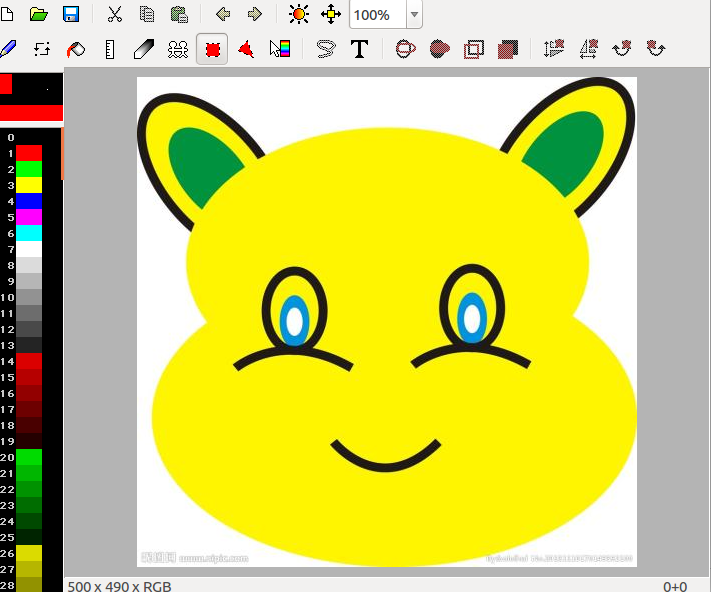展开全文• 最近在做自动泊车项目中的车位线检测，用到了将图像像素坐标转换为真实世界坐标的过程，该过程可以通过世界坐标到图像像素坐标之间的关系进行求解，在我的一篇博文中已经详细讲解了它们之间的数学关系，不清楚的童鞋...
最近在做自动泊车项目中的车位线检测，用到了将图像像素坐标转换为真实世界坐标的过程，该过程可以通过世界坐标到图像像素坐标之间的关系进行求解，在我的一篇博文中已经详细讲解了它们之间的数学关系，不清楚的童鞋们可参考:相机标定：从世界坐标系到图像像素坐标系转换过程解析
推导过程如下：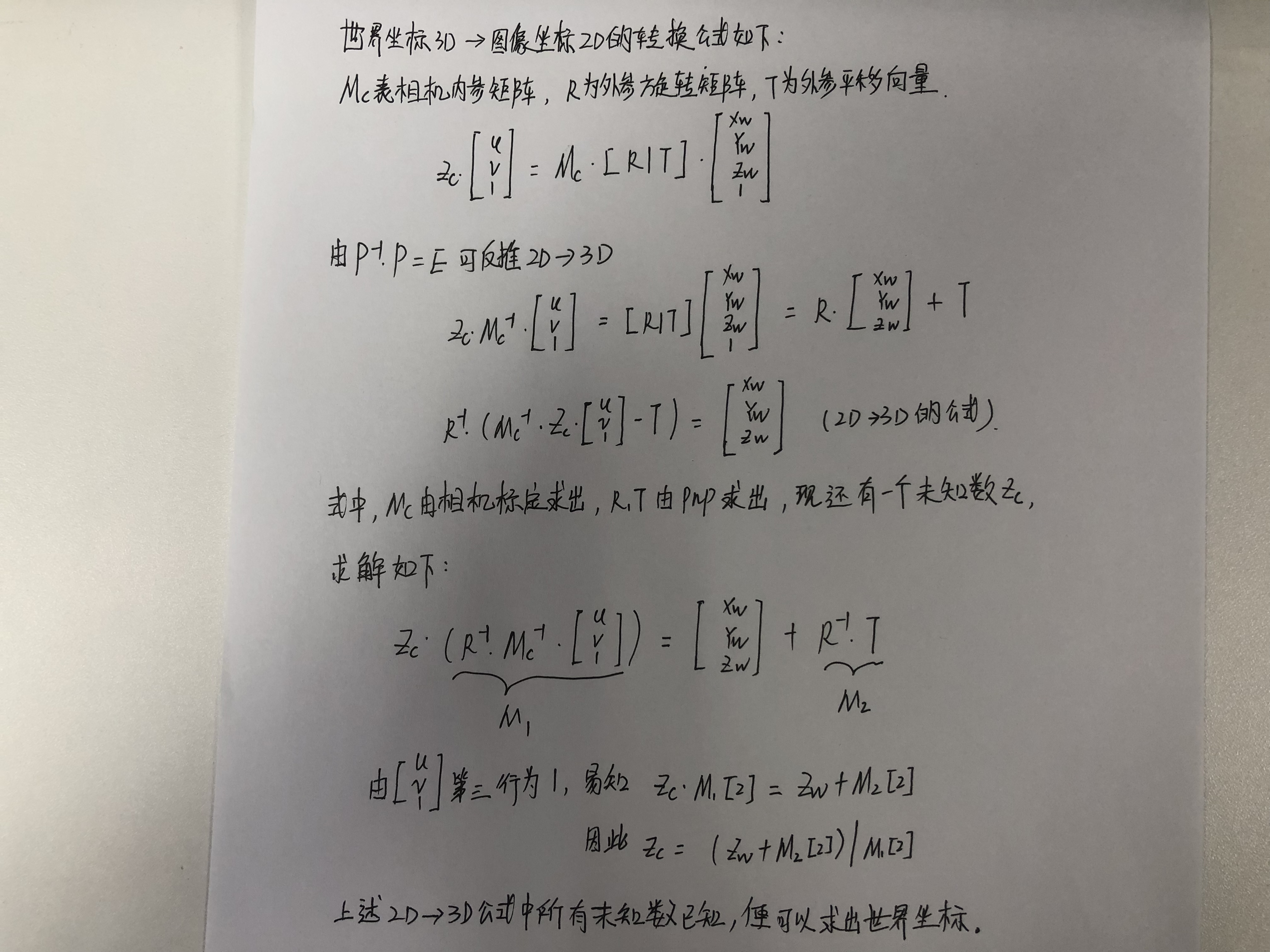一般情况下，摄像头的内参是我们事先标定好的，具体标定方法可以参考这篇博文：张正友相机标定Opencv实现以及标定流程&&标定结果评价&&图像矫正流程解析（附标定程序和棋盘图）
其次，用到了相机位姿估计中求解PNP的问题，相机位姿估计就是通过几个已知坐标的特征点，以及他们在相机照片中的成像，从而得到相机坐标系关于世界坐标系的旋转矩阵R与平移矩阵T，可以直接调用opencv::solvePnP函数求解，详细讲解可以参考这篇博文：相机位姿估计
具体标定方法如下：
1.以车身中心投影到地面的位置为世界坐标系的原点，垂直于地面朝上为z轴正向，车头方向为x轴的正向，y轴方向满足右手法则。
2.车位线识别中只用到了右侧摄像头，在水平地面上放置标定板，不要共线，记录出标定板的世界坐标（至少需要4个点）以及在图像中的像素坐标。3.调用opencv::solvePnP求解相机坐标系关于世界坐标系的旋转矩阵R与平移矩阵T。
4.根据上面公式的推倒求解出图像中车位线在世界坐标中的真实位置。
下面是实现该过程的c++/opencv的代码：
#include <opencv2/opencv.hpp>
#include <iostream>
#include <ctype.h>
using namespace cv;
using namespace std;
int main()
{
// 首先通过标定板的图像像素坐标以及对应的世界坐标，通过PnP求解相机的R&T//
Point2f point;
vector<Point2f> boxPoints; //存入像素坐标
namedWindow("Source", 1);
/ Setting box corners in image
//one Point
point = Point2f((float) 558, (float) 259); //640X480
boxPoints.push_back(point);
circle(sourceImage, boxPoints, 3, Scalar(0, 255, 0), -1, 8);

two Point
point = Point2f((float) 629, (float) 212); //640X480
boxPoints.push_back(point);
circle(sourceImage, boxPoints, 3, Scalar(0, 255, 0), -1, 8);

three Point
point = Point2f((float) 744, (float) 260); //640X480
boxPoints.push_back(point);
circle(sourceImage, boxPoints, 3, Scalar(0, 255, 0), -1, 8);

four Point
point = Point2f((float) 693, (float) 209); //640X480
boxPoints.push_back(point);
circle(sourceImage, boxPoints, 3, Scalar(0, 255, 0), -1, 8);

//Setting box corners in real world
vector<Point3f> worldBoxPoints;  //存入世界坐标
Point3f tmpPoint;
tmpPoint = Point3f((float) 2750, (float) 890, (float) 0);
worldBoxPoints.push_back(tmpPoint);
tmpPoint = Point3f((float) 3500, (float) 450, (float) 0);
worldBoxPoints.push_back(tmpPoint);
tmpPoint = Point3f((float) 2790, (float) -240, (float) 0);
worldBoxPoints.push_back(tmpPoint);
tmpPoint = Point3f((float) 3620, (float) -50, (float) 0);
worldBoxPoints.push_back(tmpPoint);
//camera  intristic///
cv::Mat cameraMatrix1=Mat::eye(3, 3, cv::DataType<double>::type);  //相机内参矩阵
cv::Mat distCoeffs1(5, 1, cv::DataType<double>::type);  //畸变参数
distCoeffs1.at<double>(0,0) = 0.061439051;
distCoeffs1.at<double>(1,0) = 0.03187556;
distCoeffs1.at<double>(2,0) = -0.00726151;
distCoeffs1.at<double>(3,0) = -0.00111799;
distCoeffs1.at<double>(4,0) = -0.00678974;

//Taken from Mastring OpenCV d
double fx = 328.61652824;
double fy = 328.56512516;
double cx = 629.80551148;
double cy = 340.5442837;
cameraMatrix1.at<double>(0, 0) = fx;
cameraMatrix1.at<double>(1, 1) = fy;
cameraMatrix1.at<double>(0, 2) = cx;
cameraMatrix1.at<double>(1, 2) = cy;

//PnP solve R&T///
cv::Mat rvec1(3, 1, cv::DataType<double>::type);  //旋转向量
cv::Mat tvec1(3, 1, cv::DataType<double>::type);  //平移向量
cv::solvePnP(worldBoxPoints, boxPoints, cameraMatrix1, distCoeffs1, rvec1, tvec1, false,CV_ITERATIVE);
cv::Mat rvecM1(3, 3, cv::DataType<double>::type);  //旋转矩阵
cv::Rodrigues(rvec1, rvecM1);

/此处用于求相机位于坐标系内的旋转角度,2D-3D的转换并不用求
const double PI=3.1415926;
double thetaZ=atan2(rvecM1.at<double>(1,0),rvecM1.at<double>(0,0))/PI*180;
double thetaY=atan2(-1*rvecM1.at<double>(2,0),sqrt(rvecM1.at<double>(2,1)*rvecM1.at<double>(2,1)
+rvecM1.at<double>(2,2)*rvecM1.at<double>(2,2)))/PI*180;
double thetaX=atan2(rvecM1.at<double>(2,1),rvecM1.at<double>(2,2))/PI*180;
cout<<"theta x  "<<thetaX<<endl<<"theta Y: "<<thetaY<<endl<<"theta Z: "<<thetaZ<<endl;

///根据公式求Zc，即s
cv::Mat imagePoint = cv::Mat::ones(3, 1, cv::DataType<double>::type);
cv::Mat tempMat, tempMat2;
//输入一个2D坐标点，便可以求出相应的s
imagePoint.at<double>(0,0)=558;
imagePoint.at<double>(1,0)=259;
double zConst = 0;//实际坐标系的距离
//计算参数s
double s;
tempMat = rvecM1.inv() * cameraMatrix1.inv() * imagePoint;
tempMat2 = rvecM1.inv() * tvec1;
s = zConst + tempMat2.at<double>(2, 0);
s /= tempMat.at<double>(2, 0);
cout<<"s : "<<s<<endl;
///3D to 2D
cv::Mat worldPoints=Mat::ones(4,1,cv::DataType<double>::type);
worldPoints.at<double>(0,0)=3620;
worldPoints.at<double>(1,0)=-590;
worldPoints.at<double>(2,0)=0;
cout<<"world Points :  "<<worldPoints<<endl;
Mat image_points=Mat::ones(3,1,cv::DataType<double>::type);
//setIdentity(image_points);
Mat RT_;
hconcat(rvecM1,tvec1,RT_);
cout<<"RT_"<<RT_<<endl;
image_points=cameraMatrix1*RT_*worldPoints;
Mat D_Points=Mat::ones(3,1,cv::DataType<double>::type);
D_Points.at<double>(0,0)=image_points.at<double>(0,0)/image_points.at<double>(2,0);
D_Points.at<double>(1,0)=image_points.at<double>(1,0)/image_points.at<double>(2,0);
//cv::projectPoints(worldPoints, rvec1, tvec1, cameraMatrix1, distCoeffs1, imagePoints);
cout<<"3D to 2D:   "<<D_Points<<endl;

//camera_coordinates
Mat camera_cordinates=-rvecM1.inv()*tvec1;
/2D to 3D///
cv::Mat imagePoint_your_know = cv::Mat::ones(3, 1, cv::DataType<double>::type); //u,v,1
imagePoint_your_know.at<double>(0, 0) = 558;
imagePoint_your_know.at<double>(1, 0) = 259;
Mat wcPoint = rvecM1.inv() * (cameraMatrix1.inv() *s*imagePoint_your_know - tvec1);
Point3f worldPoint(wcPoint.at<double>(0, 0), wcPoint.at<double>(1, 0), wcPoint.at<double>(2, 0));
cout <<"2D to 3D :"<< worldPoint << endl;
imshow("Source",sourceImage);
waitKey(0);
return 0;
}

求出各种参数以后可以将其封装为一个函数，实现图像坐标到世界坐标的转换：
Point3f getWorldPoints(Point2f inPoints)
{
cv::Mat imagePoint = cv::Mat::ones(3, 1, cv::DataType<double>::type); //u,v,1
imagePoint.at<double>(0, 0) = inPoints.x;
imagePoint.at<double>(1, 0) = inPoints.y;
Mat wcPoint = rotationMatrix.inv() * (s * cameraMatrix.inv() * imagePoint - tvec);
Point3f worldPoint(wcPoint.at<double>(0, 0), wcPoint.at<double>(1, 0), wcPoint.at<double>(2, 0));
return worldPoint;
}



展开全文• ## 得到图像像素坐标

热门讨论 2013-04-11 09:49:26
很好的VC代码 当鼠标放在打开图像的窗口上时可以得到该点的像素坐标 和大家分享一下 希望有用~~
• 图像经常使用到坐标，但是，最近发现不同的图像处理库，对坐标的定义或者标准不一致，现在总结一下 1、Python - OpenCV 库的坐标 原点为左上角(0, 0) -->(x, y) x轴为水平方向，也就是宽。 y轴为竖直方向，也...
图像经常使用到坐标，但是，最近发现不同的图像处理库，对坐标的定义或者标准不一致，现在总结一下
1、Python - OpenCV 库的坐标

原点为左上角(0, 0) -->(x, y)
x轴为水平方向，也就是宽。
y轴为竖直方向，也就是高。2、Python - PIL 库的坐标


展开全文opencv 计算机视觉 python
• 看了很多讲解针孔相机... 世界坐标系、相机坐标系、图像物理坐标系、图像像素坐标系在我的另一篇博文里已经详细叙述。http://blog.csdn.net/wangxiaokun671903/article/details/37935113。他们之间的关系如下图所示：


看了很多讲解针孔相机模型中从世界坐标系--->到相机坐标系——>图像坐标系的文章，心里的疑惑也逐渐展开，现在总结一下自己的理解：
世界坐标系、相机坐标系、图像物理坐标系、图像像素坐标系在我的另一篇博文里已经详细叙述。http://blog.csdn.net/wangxiaokun671903/article/details/37935113。他们之间的关系如下图所示：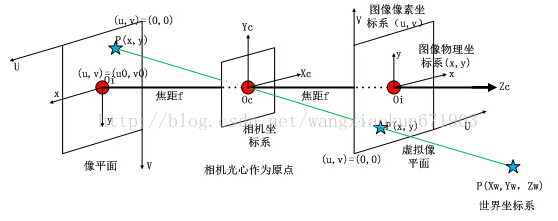从世界坐标系到图像坐标系的转换过程如下：

1、世界坐标系通过外参矩阵转换到相机坐标系
空间中一点的世界坐标系为：[Xw,Yw,Zw]T，当把它转换到相机坐标系的时候，先把它加一维用齐次坐标来表示它（这样就方便对它本身进行平移操作）。左乘一个3x4的外参矩阵，从世界纵坐标系转换到相机坐标系。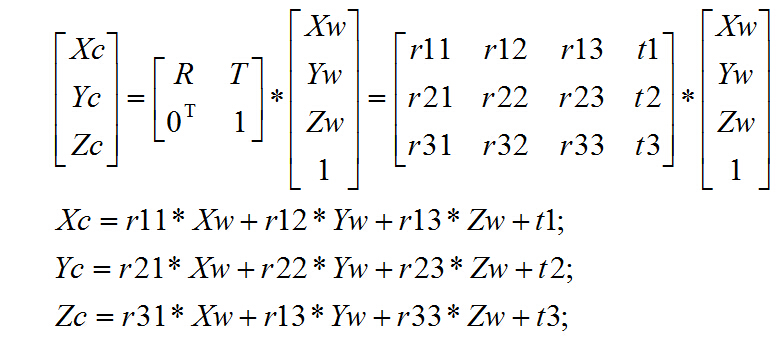[Xc,Yc,Zc]T表示相机坐标。[Xw,Yw,Zw，1]T表示归物体所在的世界坐标。R表示旋转矩阵，T表示平移矩阵。

2、相机坐标系通过内参矩阵转换到图像像素坐标系：这一步是通过两步完成的。

（1），径向畸变和切向畸变：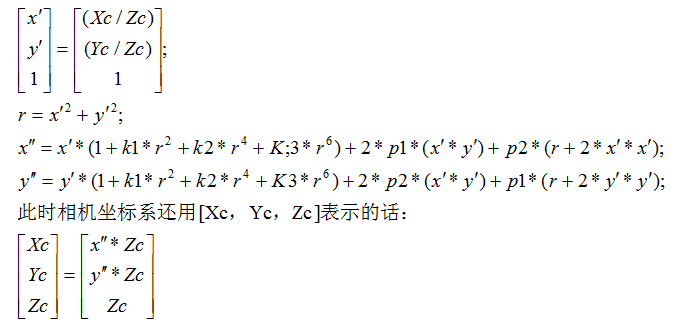其中，k1,k2,k3径向畸变系数，p1,p2是切向畸变系数。畸变发生在相机坐标系转图像物理坐标系的过程中。操作的对象时相机坐标系。
这一步是加入畸变的过程，如果忽略这一步，可以直接跳到下一步。
（2），相机坐标系通过焦距对角矩阵和畸变系数转换到图像物理坐标系；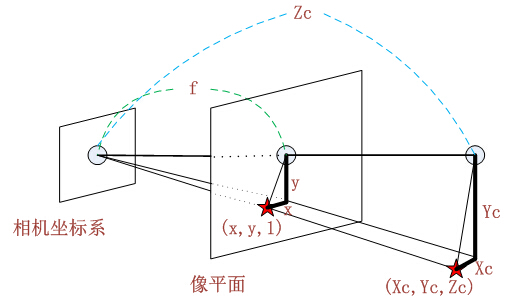由图相似三角形可得：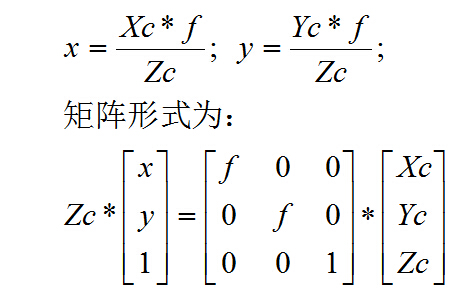f表示焦距。[Xc,Yc,Zc]T表示相机坐标。[x,y,1]T表示归一化后的图像物理坐标。

（3），图像物理坐标系通过像素转换矩阵转换到像素坐标系中。这一步是在同一个平面上做的，只不过先换了表示单位，又换了坐标原点的位置。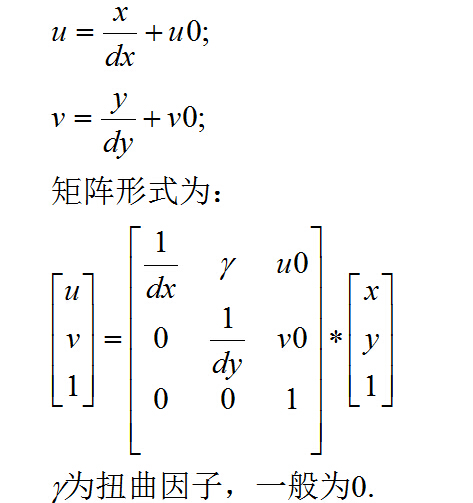dx和dy表示：x方向和y方向的一个像素分别占多少个（可是小数）长度单位。u0，v0表示图像的中心像素坐标和图像原点像素坐标之间相差的横向和纵向像素数。

3、转换过程总公式如下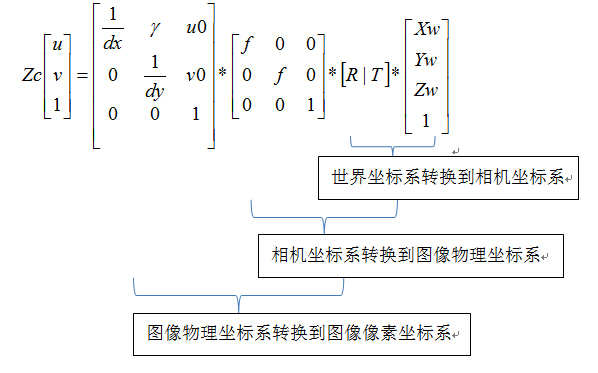展开全文• 最近师兄让我用python让我写一个遥感图像像素坐标与地理坐标进行转换的小程序，虽然比较简单，但是还要记录一下。不同坐标系的转换，涉及到仿射变换，又称仿射映射，是指在几何中，一个向量空间进行一次线性变换并...
• 最近师兄让我用python让我写一个遥感图像像素坐标与地理坐标进行转换的小程序，虽然比较简单，但是还要记录一下。不同坐标系的转换，涉及到仿射变换，又称仿射映射，是指在几何中，一个向量空间进行一次线性变换并...
• 最近在做自动泊车项目，利用视觉算法进行车位线检测，其中用到了二维图像坐标与世界坐标的相互转换，因此对相机的标定，相机的位姿计算研究了一番，抽空总结了一下，分享给广大网友。 在图像测量过程以及机器视觉...相机标定
• https://blog.csdn.net/humanking7/article/details/44756073世界坐标系
• ## matlab获取图像像素坐标[已验证]

万次阅读 多人点赞 2018-05-23 09:29:01
1. impixelinfo%例如: %1.1 显示图片 mainf=imshow(data); %show the picture %1.2 命令框中... ginput[x,y] = ginput(n)%例如: %显示图片 mainf=imshow(data); %show the picture%↓返回的x值 ↓返回的x值 ...
• 相机在计算机视觉应用中起着重要作用，作为图像数据来源，影响着后续各个处理步骤。成像模型就是用数学公式刻画整个成像过程，即被拍摄物体空间点到照片成像点之间的几何变换关系。 总体上，相机成像可以分为四个...
• 1. 像素坐标系： 如下图所示：像素坐标系u-v的原点为O0， 横坐标u和纵坐标v分别是图像所在的行和列， 在视觉处理库OpenCV中，u对应x，v对应...图像坐标系x-y的原点是O1，为像素坐标系的中点， 如图所示： 假设...
• 像素坐标系： ...则图像坐标系和像素坐标系的关系如下： 假设物理坐标系中的单位为毫米，那么dx的单位为毫米/像素。 那么x/dx的单位就是像素了， 即和u的单位一样。 为了方便， 将上式写成矩阵形式：
• 世界坐标系，相机坐标系，图像坐标系，图像像素坐标系这四个坐标系的转换实质就是刚体变换、透视投影和数字化图像这几个成像里的步骤。 一、世界坐标系到相机坐标系 世界坐标系，也称为测量坐标系，它是一个三维...世界坐标系 相机坐标系
• 世界坐标系-相机坐标系：相机坐标系相对于世界坐标系的转换矩阵 相机坐标系-图像坐标系：属于透视投影关系，真实世界中的景象投射到相机中的转换矩阵，...图像坐标系-像素坐标系：像素和图像间的比例关系 参考 ...
• 如下图，对于世界坐标系的一点P，我们需要求出其在像素坐标系的位置。 (1)世界坐标系->相机坐标系 令R,T表示世界坐标系转换到相机坐标系的旋转矩阵和平移矢量，则P在相机坐标系的坐标为 (2)相机坐标系->图像...计算机视觉 opencv python webgl
• 世界坐标、相机坐标、图像坐标、像素坐标的原理、关系，并用matlab仿真 照相机是日常生活中最常见的。它能把三维的空间图片等比例缩小投影在照片上，称为一个二维图像。 以下我们就讲一讲原理，并相应的进行matlab...世界坐标系 matlab仿真
• 世界坐标系、相机坐标系、图像坐标系、像素坐标系之间的转换 图像处理、立体视觉等方向常常涉及到四个坐标系：世界坐标系、相机坐标系、图像坐标系、像素坐标系。例如下图： 构建世界坐标系只是为了更好的描述...世界坐标系 相机坐标系
• 相机成像原理：世界坐标系、相机坐标系、图像坐标系、像素坐标系之间的转换计算机视觉 相机成像原理
• 1、世界坐标系到相机坐标系之间的转换：世界坐标系是真实世界的基准坐标系，我们需要知道相机坐标系下的点在世界坐标中的位置，利用齐次坐标转换矩阵。 2、相机坐标系到图像...3、图像最坐标系到像素坐标系： ...
• 参考博客１参考博客２世界 ——&gt; 相机 (外参：R,T ) [Xc,Yc,Zc]T表示相机坐标，[Xw,Yw,Zw，1]T表示归...此时投影点p的单位还是mm，并不是pixel，需要进一步转换到像素坐标系f表示焦距。[Xc,Yc,Zc]T表示相机...
• 设该坐标系下存在一个物体P的坐标为(X, Y, C)相机坐标系：以相机光点为中心，X，Y轴平行于图像的两条边，光轴为Z轴所建立的坐标系。用（Xc, Yc, Zc)表示物体P在相机坐标系下的位置。世界坐标系和相机坐标系的关系：...
• 如果通过api MapDepthToColorImagePoint，只能得到彩色图像像素坐标。 假如我现在得到了彩色图像像素坐标了，那我也没办法直接通过该坐标知道该像素的具体信息（RGB值）吧？因为像素的信息是存放在 frame ...kinect 深度...